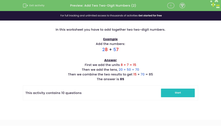### Comprehensive & curriculum aligned

In this worksheet, students add together two-digit numbers by adding the units, then adding the tens and combining the results.Key stage:  KS 2

Curriculum topic:   Number: Addition and Subtraction

Curriculum subtopic:   Use Written Addition/Subtraction to Three Digits

Popular topics:   Addition worksheets, Place Value worksheets

Difficulty level:#### Worksheet Overview

In this activity, we will learn how to add together two three-digit numbers.

Three-digit numbers have hundreds, tens and ones.

One way to add them together is to partition them into their place value.

453 + 429

453 has four hundreds, five tens, and three ones.

429 has four hundreds, two tens, and nine ones.

Once partitioned, we can add the hundreds, tens and ones.

3 + 9 = 12

50 + 20 = 70

400 + 400 = 800

Lastly, we can add together these numbers to find out the total sum.

800 + 70 + 12 = 882

Let's try an example together.

Example

628 + 257

First we add the ones: 8 + 7 = 15

Then we add the tens: 20 + 50 = 70

Then we add the hundreds: 600 + 200 = 800

Lastly, we combine the three results: 800 + 70 + 15 = 885

Now it's your turn to try some questions like this.

### What is EdPlace?

We're your National Curriculum aligned online education content provider helping each child succeed in English, maths and science from year 1 to GCSE. With an EdPlace account you’ll be able to track and measure progress, helping each child achieve their best. We build confidence and attainment by personalising each child’s learning at a level that suits them.

Get started